• 基本数据结构与算法 —（1）栈的基本操作 一.用数组实现 //定义节点结构 #define MaxSize <数组元素个数> typedef struct sNode{ ElemType data[MaxSize]; int top; }stack; //创建栈 stack* createStack...
基本数据结构与算法
（1）栈的基本操作
一.用数组实现
//定义节点结构
#define MaxSize <数组元素个数>
typedef struct sNode{
ElemType data[MaxSize];
int		 top;
}stack;

//创建栈
stack* createStack()
{
stack* s=(stack*)malloc(sizeof(stack));
s->top=-1;//数组下标为-1时代表为空栈
return s;
}

//压栈操作
void push (stack* ptrS,ElemType item)
{
if(ptrS->top==MaxSize-1)//数组下表从零开始，故判断MaxSize-1为栈满情况
{
printf("栈已满")；
return；
}
else
{
//先让头部指针向上移动，再添加数据元素
ptrS->data[++top]=item;
return;
}
}

//出栈
ElemType pop(stack* ptrS)
{
if(ptrS->top==-1)
{
printf("栈已空");
return NULL;
}
else
{
//取得数据后让top向下移
return ptrS->data[ptrS->top--];
}
}

//判断栈是否为空
bool isEmpty(stack* ptrS)
{
if(ptrS->top==-1)
return true;
else
return false;
}

//判断栈是否已满
bool isFull(stack* ptrS)
{
if(ptrS->top==MaxSize-1)
return true;
else
return false;
}

//求栈的深度
int Size(stack* ptrS)
{
return ptrS->top+1;//数组从零开始，故需加一
}

//获取栈顶元素
ElemType top(stack* ptrS)
{
if(ptrS->top==-1)//栈为空
{
return NULL;
}
return ptrS->data[top];
}


用数组实现栈时由于要预先分配后好存储空间，故在大多数时候会造成空间资源的浪费，因此为了提高空间利用率，采用两个栈各自向中间延伸的方法。

#define MaxSize <数据最大存储量>
typedef struct sNode
{
ElemType data[MaxSize];
int top1;
int top2;
}stack;
void initStack(stack* ptrS)
{
ptrS->top1=-1;
ptrS->top2=MaxSize;
}

void push(stack* ptrS,ElemType item,int tag)
{/*tag作为标志，取值为1时向第一个栈中加入数据，为2时向第二个栈中加入数据*/
if(ptrS->top2-ptrS->top1==1)
{
printf("栈已满")；
return;
}
else if(tag==1)
{
//和单一栈的操作一致
ptrS->data[++ptrS->top1]=item;
}
else if(tag==2)
{
//和单一栈的操作一致
ptrS->data[++ptrS->top2]=item;
}
}

ElemType pop(stack* ptrS,int tag)
{
if(tag==1)
{
if(ptrS->top1==-1)
{
printf("栈1已空");
return NULL;
}
else
return ptrS->data[ptrS->top1--];
}
else
{
if(ptrS->top2==MaxSize)
{
printf("栈2已满");
return NULL;
}
else
return ptrS->data[ptrS->top2--];
}
}

二.用链表实现

栈的链式存储结构实际上是一个单链表，栈的入栈与出栈只能在链表的头部进行。

//定义链表的栈节点
typedef struct sNode
{
ElemType Data;
sNode*   next;
}stack;

/*构建一个链表头指针*/
stack* createStack()
{
stack* s=(stack*)malloc(sizeof(stack));
s->next=NULL;
return s;
}

void push(stack* ptrS,ElemType item)
{
//创建新的节点
stack* tmp=(stack*)malloc(sizeof(stack));
tmp->Data=item;//将数据元素赋值给新节点的数据域
tmp->next=ptrS->next;//将新节点的指针域指向下一节点
ptrS->next=tmp;//将头节点的指针域指向新节点
}

ElemType pop(stack* ptrS)
{
/*弹栈操作*/
ElemType elem;//存储弹栈数据
stack* p=ptrS->next;//创建临时指针指向弹栈节点
ptrS->next=p->next;//让头指针指向后一个节点
elem=p->Data;
free(p);//释放掉出栈后元素对应的内存空间
return elem;
}

bool isEmpty(stack* ptrS)
{
/*判断栈是否为空*/
if(ptrS->next==NULL)
return true;
else
return false;
}



展开全文• （一） 栈定义栈是一种特殊的...栈的基本操作对于栈来说，插入数据以及删除数据都只能在栈尾进行，在栈中增加数据我们叫压栈push，删除栈中的数据我们叫出栈pop。接下来对于栈的基本操作，我们分别基于顺序栈和链...（一） 栈定义栈是一种特殊的线性表，与数组和链表的不同之处体现在增加和删除操作。具体表现在栈的读取插入和删除操作只能在栈的末尾进行。栈是一种先进后出的线性表结构，用数组存储数据的栈叫顺序栈，用链表的形式存储数据的栈我们叫链栈。栈的基本操作对于栈来说，插入数据以及删除数据都只能在栈尾进行，在栈中增加数据我们叫压栈push，删除栈中的数据我们叫出栈pop。接下来对于栈的基本操作，我们分别基于顺序栈和链栈来进行讨论。顺序栈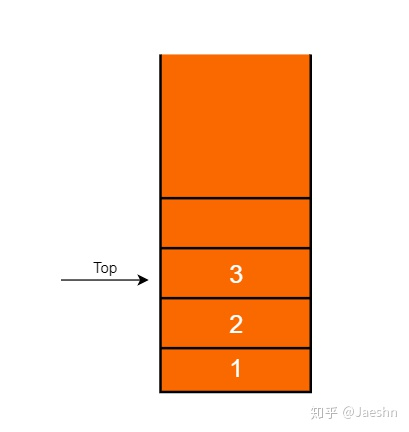查找数据：顺序栈是用数组存储数据的栈，因此对于顺序栈的查找，与数组的查找相同，如果是根据下标随机访问，那么查找的时间复杂度为O(1)；如果是查找满足特定条件的元素，需要遍历栈，那么时间复杂度为O(n)。插入数据：由于栈的特性，我们要插入数据，只能在栈的末尾进行，不过需要注意的是，由于顺序栈是使用数组存储数据的，我们在定义一个顺序栈的时候必须指定栈的大小，那么插入数据的时候需要考虑栈是否满了，如果栈还有多余的空间， 那么插入数据只需要将数据插入栈的末尾，时间复杂度为O(1)；如果栈满了，这时候需要考虑到扩容，跟数组是一样的情况，需要搬移数据，那么时间复杂度为O(n)。删除数据：删除栈中的数据，和插入数据一样只能在栈的末尾进行，不同的是，删除栈中的数据，我们不需要考虑栈是否有多余空间，不会涉及到内存申请和数据搬移，因此时间复杂度为O(1)。class 链栈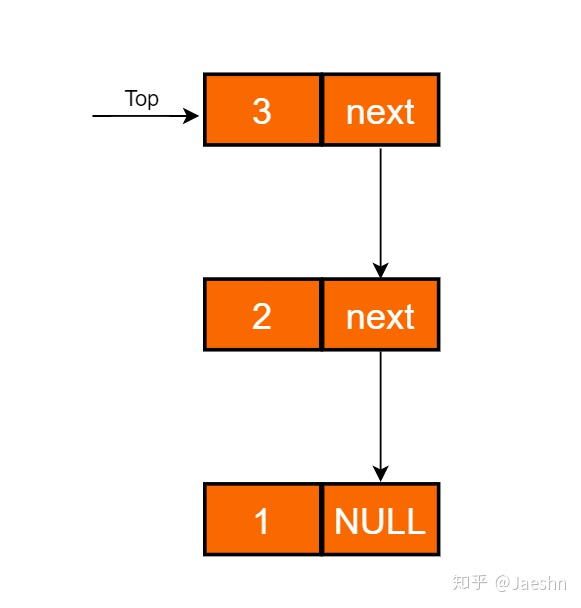查找数据：链栈是用链表存储数据的栈，与链表的查找相同，链栈的查找操作需要遍历栈，时间复杂度为O(n)。插入数据：与顺序栈不同，链栈是一个动态的线性表，在初始化一个链栈的时候不需要指定栈的大小，因此在链栈中插入数据只需要直接在链栈的末尾插入数据，时间复杂度为O(1)。删除数据：删除链栈中的数据，只需要删除链栈末尾的节点，时间复杂度为O(1)。class 应用1、括号匹配2、浏览器的前进后退功能
展开全文• 定义线性表中一种特殊数据结构，数据只能从固定一端插入数据或删除数据，另一端是封死。特点FILO(First In Last Out): 先进后出；满还存会“上溢”，空再取会“下溢”；“上溢”：在已经存满数据元素...
栈定义线性表中的一种特殊数据结构，数据只能从固定的一端插入数据或删除数据，另一端是封死的。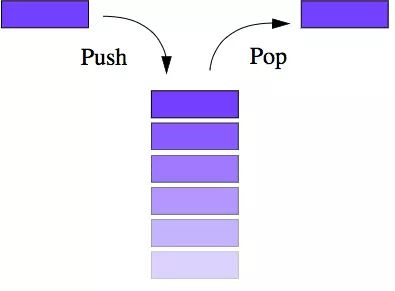特点FILO(First In Last Out): 先进后出；栈满还存会“上溢”，栈空再取会“下溢”；“上溢”：在栈已经存满数据元素的情况下，如果继续向栈内存入数据，栈存储就会出错。“下溢”：在栈内为空的状态下，如果对栈继续进行取数据的操作，就会出错。分类顺序栈特点采用数组实现，数据在物理结构上保持连续性。代码实现package one.wangwei.algorithms.datastructures.stack.impl; import one.wangwei.algorithms.datastructures.stack.IStack; import java.util.Arrays; /**  * 顺序栈  *  * @param   * @author wangwei  * @date 2018/05/04  */ public class ArrayStack implements IStack {     /**      * 默认大小      */     private static final int DEFAULT_SIZE = 10;     /**      * 数组      */     private T[] array = (T[]) new Object[DEFAULT_SIZE];     /**      * 大小      */     private int size;     /**      * 入栈      *      * @param value      * @return      */     @Override     public boolean push(T value) {         if (size >= array.length) {             grow();         }         array[size] = value;         size++;         return false;     }     /**      * 扩容50%      */     private void grow() {         int growSize = size + (size << 1);         array = Arrays.copyOf(array, growSize);     }     /**      * 压缩50%      */     private void shrink() {         int shrinkSize = size >> 1;         array = Arrays.copyOf(array, shrinkSize);     }     /**      * 出栈      *      * @return      */     @Override     public T pop() {         if (size <= 0) {             return null;         }         T element = array[--size];         array[size] = null;         int shrinkSize = array.length >> 1;         if (shrinkSize >= DEFAULT_SIZE && shrinkSize > size) {             shrink();         }         return element;     }     /**      * 查看栈顶值      *      * @return      */     @Override     public T peek() {         if (size <= 0) {             return null;         }         return array[size - 1];     }     /**      * 删除元素      *      * @param value      * @return      */     @Override     public boolean remove(T value) {         if (size <= 0) {             return false;         }         for (int i = 0; i < size; i++) {             T t = array[i];             if (value == null && t == null) {                 return remove(i);             }             if (value != null && value.equals(t)) {                 return remove(i);             }         }         return false;     }     /**      * 移除 index 处的栈值      *      * @param index      * @return      */     private boolean remove(int index) {         if (index < 0 || index >= size) {             throw new ArrayIndexOutOfBoundsException("Index: " + index + ", Size: " + size);         }         if (index != --size) {             System.arraycopy(array, index + 1, array, index, size - index);         }         array[size] = null;         int shrinkSize = array.length >> 1;         if (shrinkSize >= DEFAULT_SIZE && shrinkSize > size) {             shrink();         }         return true;     }     /**      * 清空栈      */     @Override     public void clear() {         if (size <= 0) {             return;         }         for (int i = 0; i < size; i++) {             array[i] = null;         }         size = 0;         array = null;     }     /**      * 是否包含元素      *      * @param value      * @return      */     @Override     public boolean contains(T value) {         if (size <= 0) {             return false;         }         for (int i = 0; i < size; i++) {             T t = array[i];             if (value == null && t == null) {                 return true;             }             if (value != null && value.equals(t)) {                 return true;             }         }         return false;     }     /**      * 栈大小      *      * @return      */     @Override     public int size() {         return size;     } }复杂度空间复杂度出栈和入栈的操作，只涉及一两个临时变量的存储空间，所以复杂度为O(1).时间复杂度顺序栈在出栈和入栈的操作时，最好情况时间复杂度为O(1)，当需要扩容或者缩减时，需要迁移数据，此时为最坏复杂度，为O(n). 根据摊还分析法则，它们的均摊时间复杂度还是为O(1).链表栈特点用线性表的链式结构存储，数据在物理结构上非连续代码实现package one.wangwei.algorithms.datastructures.stack.impl; import one.wangwei.algorithms.datastructures.stack.IStack; /**  * 链表栈  *  * @param   * @author wangwei  * @date 2018/05/04  */ public class LinkedStack implements IStack {     private Node top;     private int size;     public LinkedStack() {         this.top = null;         this.size = 0;     }     /**      * 入栈      *      * @param value      * @return      */     @Override     public boolean push(T value) {         Node newTop = new Node<>(value);         if (top == null) {             top = newTop;         } else {             Node oldTop = top;             top = newTop;             oldTop.above = top;             top.below = oldTop;         }         size++;         return true;     }     /**      * 出栈      *      * @return      */     @Override     public T pop() {         if (top == null) {             return null;         }         Node needTop = top;         top = needTop.below;         if (top != null) {             top.above = null;         }         T needValue = needTop.element;         needTop = null;         size--;         return needValue;     }     /**      * 查看栈顶值      *      * @return      */     @Override     public T peek() {         return top == null ? null : top.element;     }     /**      * 删除元素      *      * @param value      * @return      */     @Override     public boolean remove(T value) {         if (top == null) {             return false;         }         Node x = top;         if (value == null) {             while (x != null && x.element != null) {                 x = x.below;             }         } else {             while (x != null && !value.equals(x.element)) {                 x = x.below;             }         }         return remove(x);     }     /**      * 删除一个节点      *      * @param node      * @return      */     private boolean remove(Node node) {         if (node == null) {             return false;         }         Node above = node.above;         Node below = node.below;         // 删除中间元素         if (above != null && below != null) {             above.below = below;             below.above = above;         }         // 删除top元素         else if (above == null && below != null) {             top = below;             top.above = null;         } else if (above != null && below == null) {             above.below = null;             below = null;         } else {             top = null;         }         node = null;         size--;         return true;     }     /**      * 清空栈      */     @Override     public void clear() {         if (top == null) {             return;         }         for (Node x = top; x != null; ) {             Node below = x.below;             x.element = null;             x.above = null;             x.below = null;             x = below;         }         top = null;         size = 0;     }     /**      * 是否包含元素      *      * @param value      * @return      */     @Override     public boolean contains(T value) {         if (value == null) {             for (Node x = top; x != null; x = x.below) {                 if (x.element == null) {                     return true;                 }             }         } else {             for (Node x = top; x != null; x = x.below) {                 if (x.element.equals(value)) {                     return true;                 }             }         }         return false;     }     /**      * 栈大小      *      * @return      */     @Override     public int size() {         return size;     }     /**      * 节点      *      * @param       */     private static class Node {         private T element;         private Node above;         private Node below;         public Node(T element) {             this.element = element;         }     } }复杂度空间复杂度出栈和入栈的操作，只涉及一两个临时变量的存储空间，所以复杂度为O(1).时间复杂度出栈和入栈的操作，不涉及数据搬迁，只是顶部元素操作，时间复杂度均为O(1).栈的应用接下来，我们看看栈在软件工程中的实际应用。函数调用操作系统给每个线程分配了一块独立的内存空间，这块内存被组织成“栈”这种结构, 用来存储函数调用时的临时变量。每进入一个函数，就会将临时变量作为一个栈帧入栈，当被调用函数执行完成，返回之后，将这个函数对应的栈帧出栈。示例：int main() {    int a = 1;     int ret = 0;    int res = 0;    ret = add(3, 5);    res = a + ret;    printf("%d", res);    reuturn 0; } int add(int x, int y) {    int sum = 0;    sum = x + y;    return sum; } 复制代码main() 函数调用了 add() 函数，获取计算结果，并且与临时变量 a 相加，最后打印 res 的值。这个过程的中函数栈里的出栈、入栈操作，如下所示：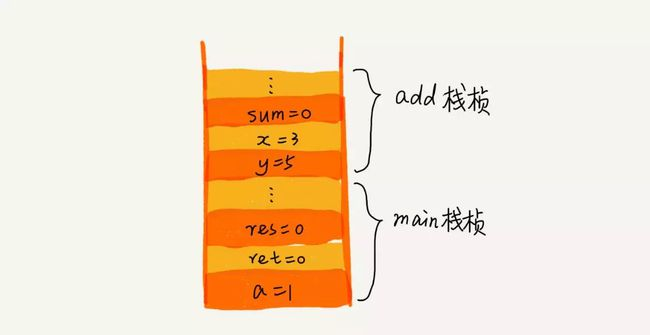思考：为什么函数要用栈来保存临时变量呢？用其他数据结构不行吗？函数调用的局部状态之所以用栈来记录是因为这些状态数据的存活时间满足“后入先出”(LIFO)顺序，而栈的基本操作正好就是支持这种顺序的访问。栈是程序设计中的一种经典数据结构，每个程序都拥有自己的程序栈。栈帧也叫过程活动记录，是编译器用来实现函数调用过程的一种数据结构。C语言中，每个栈帧对应着一个未运行完的函数。从逻辑上讲，栈帧就是一个函数执行的环境：函数调用框架、函数参数、函数的局部变量、函数执行完后返回到哪里等等。栈是从高地址向低地址延伸的。每个函数的每次调用，都有它自己独立的一个栈帧，这个栈帧中维持着所需要的各种信息。寄存器ebp(base pointer)指向当前的栈帧的底部(高地址)，可称为“帧指针”或“基址指针”；寄存器esp(stack pointer)指向当前的栈帧的顶部(低地址)，可称为“ 栈指针”。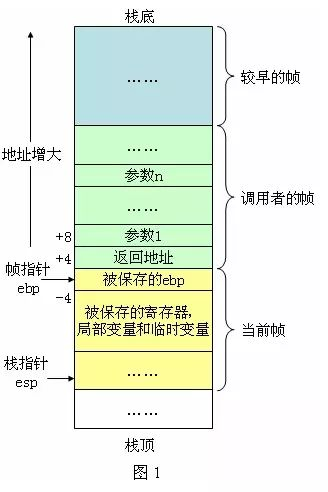在C和C++语言中，临时变量分配在栈中，临时变量拥有函数级的生命周期，即“在当前函数中有效，在函数外无效”。这种现象就是函数调用过程中的参数压栈，堆栈平衡所带来的。堆栈平衡是指函数调完成后，要返还所有使用过的栈空间。函数调用其实可以看做4个过程：1. 压栈: 函数参数压栈，返回地址压栈2. 跳转: 跳转到函数所在代码处执行3. 执行: 执行函数代码4. 返回: 平衡堆栈，找出之前的返回地址，跳转回之前的调用点之后，完成函数调用表达式求值以  3 + 5 x 8 - 6 为这个表达式为例，编译器是如何利用栈来实现表达式求值的呢？编译器会使用两个栈来实现，一个栈用来保存操作数，另一个栈用来保存运算符。从左向右遍历表达式，遇到数字直接压入操作数栈，遇到操作符，就与运算符栈顶元素进行比较。如果比运算符栈顶元素的优先级高，就将当前运算符压入栈；如果比运算符栈顶元素的优先级低或者相同，从运算符栈中取栈顶运算符，从操作数栈的栈顶取 2 个操作数，然后进行计算，再把计算完的结果压入操作数栈，继续比较。如图所示：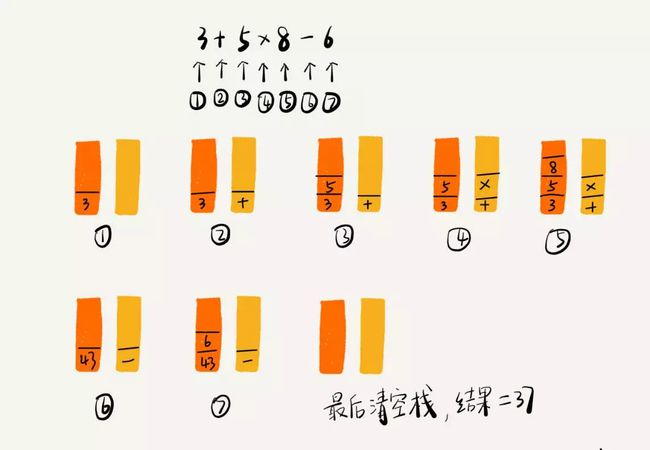此前，我们在讲 比特币脚本语言 时，提到过 逆波兰表示法 ，也是运用了栈这种数据结构特征。括号匹配栈还可以用来检测表达式中的括号是否匹配。我们假设表达式中只包含三种括号，圆括号 ()、方括号 [] 和花括号{}，并且它们可以任意嵌套。比如，{[{}]}或 [{()}([])] 等都为合法格式，而{[}()] 或 [({)] 为不合法的格式。那我现在给你一个包含三种括号的表达式字符串，如何检查它是否合法呢？我们用栈来保存未匹配的左括号，从左到右依次扫描字符串。当扫描到左括号时，则将其压入栈中；当扫描到右括号时，从栈顶取出一个左括号。如果能够匹配，比如“(”跟“)”匹配，“[”跟“]”匹配，“{”跟“}”匹配，则继续扫描剩下的字符串。如果扫描的过程中，遇到不能配对的右括号，或者栈中没有数据，则说明为非法格式。当所有的括号都扫描完成之后，如果栈为空，则说明字符串为合法格式；否则，说明有未匹配的左括号，为非法格式。浏览器的前进、后退功能使用两个栈，X 和 Y，把首次浏览的页面依次压入栈 X，当点击后退按钮时，再依次从栈 X 中出栈，并将出栈的数据依次放入栈 Y。当我们点击前进按钮时，我们依次从栈 Y 中取出数据，放入栈 X 中。当栈 X 中没有数据时，那就说明没有页面可以继续后退浏览了。当栈 Y 中没有数据，那就说明没有页面可以点击前进按钮浏览了。比如你顺序查看了 a，b，c 三个页面，我们就依次把 a，b，c 压入栈，这个时候，两个栈的数据就是这个样子：当你通过浏览器的后退按钮，从页面 c 后退到页面 a 之后，我们就依次把 c 和 b 从栈 X 中弹出，并且依次放入到栈 Y。这个时候，两个栈的数据就是这个样子：这个时候你又想看页面 b，于是你又点击前进按钮回到 b 页面，我们就把 b 再从栈 Y 中出栈，放入栈 X 中。此时两个栈的数据是这个样子：这个时候，你通过页面 b 又跳转到新的页面 d 了，页面 c 就无法再通过前进、后退按钮重复查看了，所以需要清空栈 Y。此时两个栈的数据这个样子：当然，我们还可以使用双向链表来实现这个功能。区别我们都知道，JVM 内存管理中有个“堆栈”的概念。栈内存用来存储局部变量和方法调用，堆内存用来存储 Java 中的对象。那 JVM 里面的“栈”跟本篇说的“栈”是不是一回事呢？如果不是，那它为什么又叫作“栈”呢？本篇介绍的栈是一种抽象的数据结构，而JVM中的"堆栈"是一种实际存在的物理结构。总结在此我向大家推荐一个架构学习交流群。交流学习群号：938837867 暗号：555 里面会分享一些资深架构师录制的视频录像：有Spring，MyBatis，Netty源码分析，高并发、高性能、分布式、微服务架构的原理，JVM性能优化、分布式架构等这些成为架构师必备
展开全文• 数据结构与算法C语言实现——栈的基本操作 雨中漫步 问题：编写程序任意输入栈长度和栈中的元素值，构造一个顺序栈，对其进行清空、销毁、入栈、出栈以及取栈顶元素操作。 #include <stdio.h> #include <...
数据结构与算法C语言实现——栈的基本操作
雨中漫步
问题：编写程序任意输入栈长度和栈中的元素值，构造一个顺序栈，对其进行清空、销毁、入栈、出栈以及取栈顶元素操作。
#include <stdio.h>
#include <stdlib.h>
#define MaxSize 100
typedef int ElemType;
typedef struct
{
ElemType data[MaxSize];
int top;				//栈指针
} SqStack;					//顺序栈类型
void InitStack(SqStack *&s)  //清空，初始化
{
s=(SqStack *)malloc(sizeof(SqStack));
s->top=-1;
}
void DestroyStack(SqStack *&s)  //销毁
{
free(s);
}

bool Push(SqStack *&s,ElemType e)  //入栈
{
if (s->top==MaxSize-1)    //栈满的情况，即栈上溢出
return false;
s->top++;
s->data[s->top]=e;
return true;
}
bool Pop(SqStack *&s,ElemType &e)
{
if (s->top==-1)		//栈为空的情况，即栈下溢出
return false;
e=s->data[s->top];
s->top--;
return true;
}
bool GetTop(SqStack *s,ElemType &e)
{
if (s->top==-1) 		//栈为空的情况，即栈下溢出
return false;
e=s->data[s->top];
return true;
}
int main()
{
SqStack *s;
InitStack(s);
int L,e,a,n;
printf("请输入栈长和栈的元素值\n");
scanf("%d",&L);
while(L--)
{
scanf("%d",&e);
Push(s,e);
}
n=20;
while(n--)
{
printf("清空请按1，销毁请按2，进栈请按3，出栈请按4，取栈顶元素请按5,结束请按6\n");
scanf("%d",&a);
if(a==1)
{
InitStack(s);
printf("清空成功！\n");
}
if(a==2)
{
DestroyStack(s);
printf("销毁成功！\n");
}
if(a==3)
{
printf("请输入进栈元素\n");
scanf("%d",&e);
if(Push(s,e))
printf("进栈成功！\n");
}
if(a==4)
{
if(Pop(s,e))
printf("出栈成功！\n");
}
if(a==5)
{
if(GetTop(s,e))
printf("成功！取出元素为：%d\n",e);
}
if(a==6)
{
break;
}
}

}




展开全文• 1.顺序栈的初始化 Statue InitStack(SqStack &S){ //构造一个空栈 S.base=new SElemType[MAXSIZE]; if(!S.base) exit(OVERFLOW)； //存储分配失败 S.top=S.base; //栈顶指针等于栈底指针 S.stacksize=...
• 一.栈模型 栈(Stack)又称后进先出表(LIFO List),是插入/删除操作被限制为只能在1个位置上进行的表...对栈的基本操作包括进栈(Push;在末端插入)和出栈(Pop;删除尾元素) 二.栈的实现 1.单链表实现: 2.数组实现: ...
• 一、栈的定义和特点 1) 栈是一个特殊的线性表，是限定仅在表尾(栈顶)进行插入和删除操作的线性表 ... 5) 栈的逻辑结构: 线性表相同，仍为一对一关系 6) 栈的存储结构:顺序存储或链式存储 ...
• 第三章栈和队列;...栈的抽象数据类型 ADT Stack {数据对象:D ={a ia i ElemSet, i= 1,2,n, n0} 数据关系: R = {, a i > a i-1 , a i D, i = 2,n } 约定a n端为栈顶, a 1端为栈底. 基本操作: ;StackLeng
• 数据结构与算法设计》实验报告书之栈的存储结构定义及基本操作 实验项目 栈的存储结构定义及基本操作 实验目的 . 掌握栈的逻辑特征 . 掌握栈顺序存储结构的特点，熟练掌握顺序栈的基本运算 . 熟练掌握栈的链式存储...
• 数组实现 //用数组实现 public class ArrayStackDemo { public static void main(String[] args) { ArrayStack stack = new ArrayStack(5); stack.push(1); stack.push(4); stack.push(6); stack.push(3);...链表 java
• 本篇代码是基于数据结构栈的基本操作以及实现来进行编写的，由于只是当做练习来加深理解，所以没有过多地考虑程序的健壮性。不仅有顺序栈还有链栈，以及栈在递归中的作用，像斐波那契以及hanoi塔问题的算法都用到...
• 数据结构与算法—栈栈的基本概念栈的基本API栈的常见算法括号匹配问题(LeetCode20)删除字符串中的所有相邻重复项(LeetCode1047) 栈的基本概念 栈是一种先进后出(FILO)的数据结构，是一种只能在一端进行插入和删除......

# 数据结构与算法栈的基本操作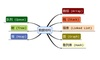数据结构 订阅